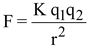# Properties of Electric Charge

## COULOMB'S LAW:

The force between two charges is directly proportional to the product of two charges (q1 and q2 )and inversely proportional to the square of distance (r) between them, where K is constant of proportionality.

(a) Electric charges can neither be destroyed nor be created.

(b) Charges are additive i.e. total charge is the algebraic sum of the individual charges.